Function Repository Resource:

# SolarAzimuthTime

Compute the time when the Sun passes a certain azimuth

Contributed by: Sander Huisman
 ResourceFunction["SolarAzimuthTime"][loc, date, azimuth] calculates at what point on the day date the sun has the azimuth azimuth at the location loc.

## Details

The calculation is independent of the altitude ("height") of the sun. Therefore it can be that the sun is below the horizon in the calculation.
Location loc can be specified as an Entity object, assuming it represents an object with geographic coordinates, or it can be a latitude/longitude pair, assuming degrees as units.
The azimuth azimuth should be in degrees.

## Examples

### Basic Examples (1)

Determine when the facade of the author's building gets sunlight on July 2nd, 2023:

 In:=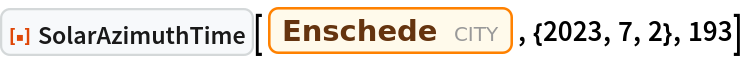Out=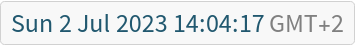### Applications (3)

Compare the time when the sun is exactly south for Amsterdam and 100 km east of Amsterdam:

 In:=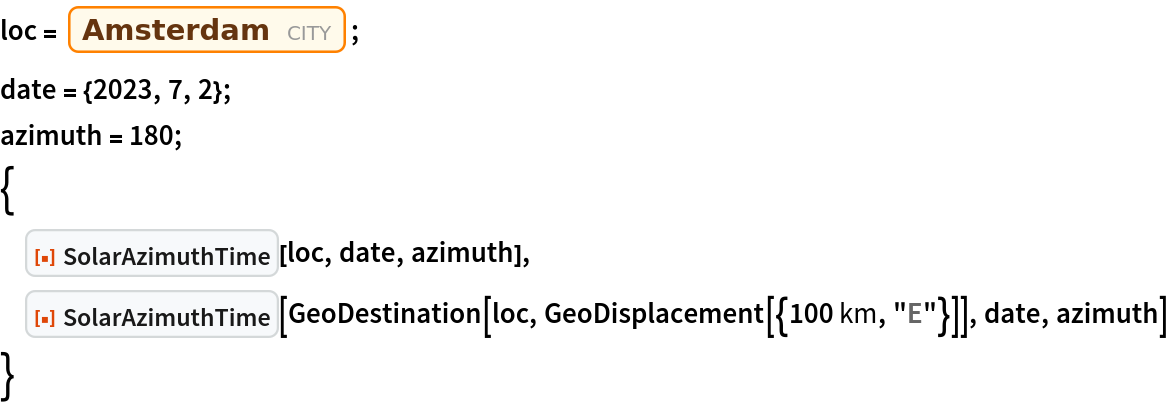Out=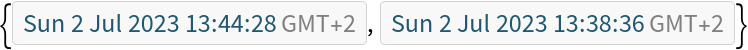Calculate the difference:

 In:=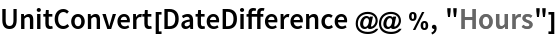Out=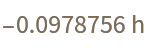This is roughly what you expect for this latitude:

 In:=Out=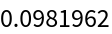### Possible Issues (1)

Though the sun might have a certain azimuth, it does not mean that the sun is above the horizon. This especially holds for places that have periods of extended darkness:

 In:=Out=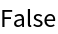### Neat Examples (1)

Plot the yearly variation of the time when the sun is perfectly south in Paris:

 In:=Out=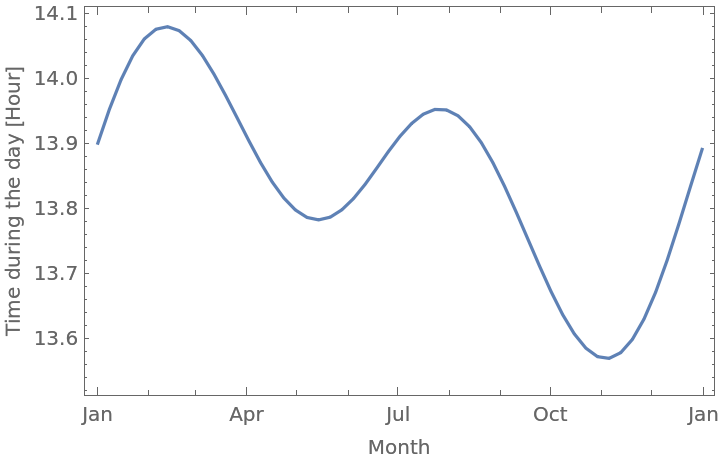SHuisman

## Requirements

Wolfram Language 13.0 (December 2021) or above

## Version History

• 1.0.0 – 13 July 2023